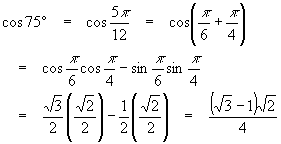Elementary Trigonometry Identities

With only the angles and their trig functions found previously, a large number of trig functions of other angles can be found with the use of a small collection of trig identities (also known as laws). An identity is an equation that is always true, so for example, the Pythagorean theoremwhere x and y are the length of the sides, and r is the length of the hypotenuse of a right triangle, is always true. Thus this equation is an identity. There are equations that are not identities. For example, the equationdescribes variables which are multiplicative inverses of each other; the equation is meaningless at x = 0, and so is not an identity.

Although there are many, many trig identities, we’ll concentrate on the use of just a few of the more useful ones, since the more obscure identities are always derived from the simpler ones. We’ll also make an effort so show how to derive these identities, in order to get some practice using algebra to assist our study of trig.

This says the sum of the squares of the sides of a right triangle equals the square of the hypotenuse. Using the unit circle (where x = cosq, y = sinq and r = 1), this becomes(Note on notation: the squares on the right hand side really apply to the entire function:It is customary and much faster to write the powers of trig functions this way, and causes no confusion one the convention is known.)

Clearly, x and y (and so, by definition, cosq and sinq) must both be between –1 and +1:Were these not true, the sum of the squares would exceed 1, and our identity would fail.

This identity is very useful, since we can find a cosine of an angle given the sine and vice-versa.

Example

Given that sin(p/6) = sin 30o = ½, find the cosine.

Solution

Solving the equation for cosq,Since we are in the first quadrant, cos(p/6) >0 and we can drop the +/-. Thus,Example

If sin 45o = cos 45o, find the value of both.

Solution

According to the identity, if we set a = sin 45o = cos 45o, we must haveand since the angle is again in the first quadrant,Without proof, the identity for the sine of a sum is given by:Sine addition formula

Example

Find sin 75o = sin (5p/12) given the above information.

Solution

Since 75 = 30 + 45, we are trying to findWe can modify the above formula to handle subtraction by replacing –y for y:Yielding:Sine subtraction formula

Example

Find sin 15o = sin(p/12) using the above data.

Solution

Since 15 = 45 – 30, we should findNote that sometimes degrees are more convenient to use than radians in planning these calculations, although radians are often better when computing the sines and cosines.

The corresponding formulae for calculating the cosines of a sum and difference are, respectively:Cosine addition formulaCosine subtraction formula

Example

Find the cosines of the angles from the two examples above.

Solution

Using the same ideas and applying the last two formulas,andThe attentive reader might have noticed that we found the trig functions for complementary angles, so that the numbers above verify that sin 15o = cos 75o and cos 15o = sin 75o !